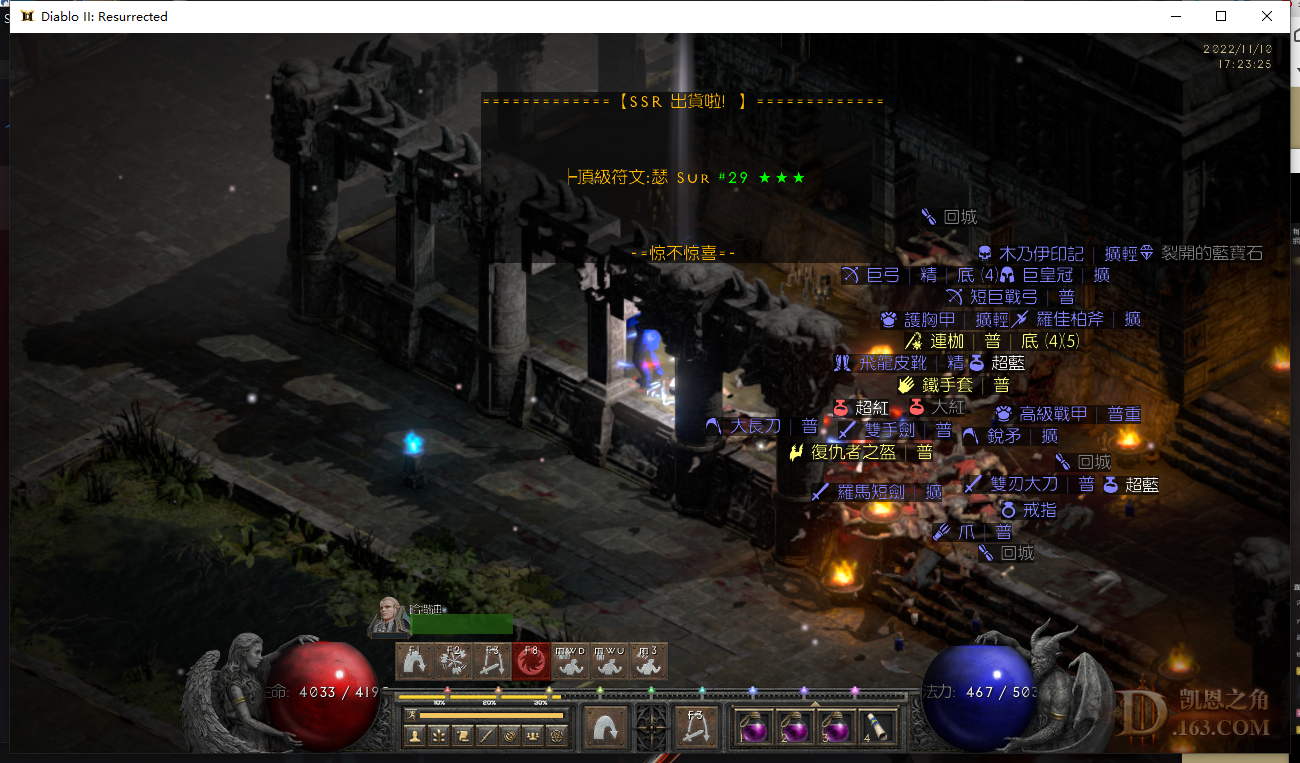//没登陆

 场次 所在楼 出货亮点 1-100 1 12fhr电板子 101-200 11 毫无亮点 201-300 13 7ll187ed无形tt 301-400 20 28# 34 371 悔恨 401-500 23 30# 女祭司随手出 501-600 24 毫无亮点 601-700 26 2x22# 701-800 28 23# 801-900 29 21# 20# 901-1000 30 25# 战斗板

33=0  32=0
31=1 牛场
30=1 3c
29=0
28=1 3c
27=1 超市 442cta
26=2 超市
25=2 cs

 1-100 0 1 2 3 4 5 6 7 8 9 0 0 gc 电加12fhr 10 23# 20 30 0 22# 40 50 22敏3孔头 60 0 70 0 80 0 90

33=0  32=0
31=1 牛场
30=1 3c
29=0
28=1 3c
27=1 超市 442cta
26=2 超市
25=2 cs

 大哥，求一下加点跟配装。。。我也想搞一个玩

 挖了几天3C，最好的就是+5-5电珠，赌了几个垃圾头盔

 牛叉，一千起步

 然后呢 6个小时过去了还是一个表格 新人学习一下

 本帖最后由 赶紧去做事 于 2022-11-10 17:48 编辑 乐散人 发表于 2022-11-10 10:10 大哥，求一下加点跟配装。。。我也想搞一个玩 现在装备都是过度 cb头 cb鞋 按手礼 杜壳  双吸戒指 反正先保证肉度，不被冰冻，和输出。武器现在是两把誓约。副手一把烧火棍 一把阿里巴巴。加点就是挖东西点满  点到70概率 剑专精点满 旋风点10点  吼点几点 我现在才80级 对了还有那个狂暴之际点一点 破物免

33=0  32=0
31=1 牛场
30=1 3c
29=0
28=1 3c
27=1 超市 442cta
26=2 超市
25=2 cs

 千万111 发表于 2022-11-10 10:14 挖了几天3C，最好的就是+5-5电珠，赌了几个垃圾头盔 换个玩法 冰法bug超市玩腻了 而且不出大号符文 我现在赌戒指

33=0  32=0
31=1 牛场
30=1 3c
29=0
28=1 3c
27=1 超市 442cta
26=2 超市
25=2 cs蛮子刚刚出了个29

2赛季符文全家桶还差33#

15冰头*4
18/12权冠15/11
28/40/40次元
15IAS 15MAX一个
11月10日晚上8点 20/20/5
 我就是老萌新 发表于 2022-11-10 18:02 蛮子刚刚出了个29 牛啊 蛮子挖3c我还是很看好的

33=0  32=0
31=1 牛场
30=1 3c
29=0
28=1 3c
27=1 超市 442cta
26=2 超市
25=2 cs

 101-200 0 1 2 3 4 5 6 7 8 9 0 0 10 0 20 0 30 40 50 0 60 70 0 80 90 0

33=0  32=0
31=1 牛场
30=1 3c
29=0
28=1 3c
27=1 超市 442cta
26=2 超市
25=2 cs

 小心被封，建议别一直3c

 201-300 0 1 2 3 4 5 6 7 8 9 0 10 0 20 0 30 0 40 50 0 60 0 70 7ll187ed无形tt 80 90 0

33=0  32=0
31=1 牛场
30=1 3c
29=0
28=1 3c
27=1 超市 442cta
26=2 超市
25=2 cs

 szwmaya 发表于 2022-11-11 18:41 是不是打钱不能有冰伤害啊，我经常把怪打碎了，是不是因为我用了执法者？ 是的，建议悔恨